# PERIODIC_BOUNDARY_CONDITION

Specifies general periodic boundary conditions on pairs of nodes.

AcuSolve Command

## Syntax

PERIODIC_BOUNDARY_CONDITION("name") {parameters...}

User-given name.

## Parameters

variable or var (enumerated) [=all]
Periodic boundary condition variable.
all
All variables. May be used only with periodic, axisymmetric and two_reference_frames types.
velocity or vel
Velocity vector.
pressure or pres
Pressure.
temperature or temp
Temperature.
eddy_viscosity or eddy
Turbulence kinematic eddy viscosity.
kinetic_energy or tke
Turbulence kinetic energy.
eddy_frequency or tomega
Turbulence eddy frequency.
dissipation_rate
Turbulence dissipation rate.
intermittency
Transition intermittency.
transition_re_theta
Transition Re-Theta.
species_1 or spec1
Species 1.
species_2 or spec2
Species 2.
species_3 or spec3
Species 3.
species_4 or spec4
Species 4.
species_5 or spec5
Species 5.
species_6 or spec6
Species 6.
species_7 or spec7
Species 7.
species_8 or spec8
Species 8.
species_9 or spec9
Species 9.
viscoelastic_stress or vest
Viscoelastic stresses.
field
Volume fraction of field in multi field for levelset and algebraic Eulerian problems or mass fraction of field in multi field for the humid air model.
mesh_displacement or mesh_disp
Mesh displacement vector.
precedence (integer) [=1]
Precedence of this boundary condition set with respect to other sets. The set with the highest value has precedence.
type (enumerated) [=periodic]
Type of the periodic boundary condition.
periodic or zero
Simple periodic condition.
axisymmetric
Axisymmetric condition. Requires rotation_axis.
two_reference_frames
Interface condition between two regions with different reference frames. Requires rotation_force_1 and rotation_force_2. Obsolete parameter.
constant_offset
Periodic condition with a constant offset. Requires constant_value for scalar variables and constant_values for vector variables.
nodal_pair_offset
Periodic condition with offset given on a nodal-pair basis. Requires nodal_pair_values.
user_function_offset
Periodic condition with offset given by a user-defined function. Requires user_function, user_values and user_strings.
constant_coefficients
General two-node constraint with constant coefficients. Requires constant_values.
nodal_pair_coefficients
General two-node constraint with coefficients given on a nodal-pair basis. Requires nodal_pair_values.
user_function_coefficients
General two-node constraint with coefficients given by a user-defined function. Requires user_function, user_values and user_strings.
single_unknown_offset
Periodic condition with a single unknown offset.
single_unknown_ratio
Periodic condition with a single unknown ratio.
nodal_pairs or pairs (array) [no default]
List of nodal point pairs in this set.
rotation_axis or axis (array) [={0,0,0;0,0,1}]
Axis of rotation. Used with axisymmetric type.
rotation_force_1 or rot_1 (string) [=none]
User-given name of the rotational force command of the first set of nodes. If none, stationary frame of reference is assumed. Used with two_reference_frames type. Obsolete parameter.
rotation_force_2 or rot_2 (string) [=none]
User-given name of the rotational force command of the second set of nodes. If none, stationary frame of reference is assumed. Used with two_reference_frames type. Obsolete parameter.
constant_value or value (real) [=0]
Constant value of offset. Used with constant_offset type for scalar variables.
constant_values or values (array) [={0,0,0}]
Constant values of offset or the constant coefficients. Used with constant_offset type for vector variables and with constant_coefficients type.
field (string) [no default]
Value of the periodic boundary condition for field. Used with variable field.
nodal_pair_values (array) [no default]
Constraint values for nodal-pairs. Used with nodal_pair_offset and nodal_pair_coefficients types.
user_function or user (string) [no default]
Name of the user-defined function. Used with user_function_offset and user_function_coefficients types.
user_values (array) [={}]
Array of values to be passed to the user-defined function. Used with user_function_offset and user_function_coefficients types.
user_strings (list) [={}]
Array of strings to be passed to the user-defined function. Used with user_function_offset and user_function_coefficients types.
multiplier_function (string) [=none]
User-given name of the multiplier function for scaling the offset values of the periodic boundary condition. If none, no scaling is performed.
active_type (enumerated) [=all]
Type of the active flag. Determines which nodal pairs in this set will have this periodic boundary condition imposed.
all
All nodal pairs in this set are active.
none
No nodal pair in this set are active.
user_function or user
User-defined function. Requires active_user_function, active_user_values and active_user_strings.
active_user_function (string) [no default]
Name of the user-defined function for the active flag. Used with user_function active type.
active_user_values (array) [={}]
Array of values to be passed to the active flag user-defined function. Used with user_function active type.
active_user_strings (list) [={}]
Array of strings to be passed to the active flag user-defined function. Used with user_function active type.

## Description

This command specifies generalized periodic boundary conditions for a solution variable (or all variables) on a set of nodal pairs. For example,
PERIODIC_BOUNDARY_CONDITION( "inflow/outflow periodicity" ) {
variable     = all
type         = periodic
nodal_pairs  = { 1, 101, 201 ;
2, 102, 202 ;
3, 103, 203 ; }
}

defines a simple periodic boundary condition on all solution fields of the problem. This command specifies that the solution at node 101 is equal to that of node 201, and similarly that the solution of node 102 is equal to that of node 202, and so on.

The variable parameter specifies the solution field to which the periodic boundary condition applies. If the problem does not solve for such a variable, the periodic boundary condition is ignored. See the STAGGER command. The all variable may be used to specify periodic boundary condition on all variables in the problem. This variable is only allowed with types periodic, axisymmetric, and two_reference_frames. A periodic boundary condition on a scalar variable, such as temperature, defines a single constraint. However, for vector variables, such as velocity, a periodic boundary condition imposes three constraints, typically in the x, y, and z directions.

The nodal_pairs parameter is a three-column array corresponding to a unique, within this set, nodal-pair number, and node one and two of the pair, one pair per row. The node numbers must be valid numbers, as given by the COORDINATE command. AcuSolve properly handles duplicate node pairs and nodes periodic with themselves.

The nodal pairs may be read from a file. For the above example, the pairs may be placed in a file, such as inflow-outflow.pbc:
1      101          201
2      102          202
2      103          203
PERIODIC_BOUNDARY_CONDITION( "inflow/outflow periodicity" ) {
variable     = all
type         = periodic
}

For a given variable, a given node may be included in more than one periodic boundary condition pair. In this case, enough periodic conditions must be supplied to uniquely specify the entire condition set. These conditions may be imposed via a single or multiple PERIODIC_BOUNDARY_CONDITION commands. For example, nodal pairs (1,2) and (3,4) may have simple periodic conditions, and nodal pairs (1,3) and (2,4) have axisymmetric conditions, with the same axis of rotation. Note that this is an over-specification of constraints (but consistent).

Any potential inconsistency in multiply-specified periodic boundary conditions on a given node is resolved through precedence. The constraints with higher values of precedence take precedence over constraints with lower values. For constraints with the same precedence value, the periodic boundary condition sets specified later take precedence over the ones specified earlier. In all cases, nodal boundary conditions take precedence over periodic boundary conditions.

A periodic type periodic boundary condition specifies that the values of a variable on two nodes are equal, as in the above example. In case of a vector variable, each component of the vector on the two nodes are equal.

An axisymmetric periodic boundary condition specifies that a vector field of two nodes be equal in a rotated coordinate system defined by an axis of rotation. The axis of rotation is given by rotation_axis. This parameter is a 3x2 array defining two points, p1 and p2, on the axis of rotation. The points are defined in the global xyz coordinate system. This provides a cylindrical coordinate system for each node of a nodal pair, n1 and n2 , where a, r and θ are the axial, radial and tangential directions, respectively; see the image below.
Let u1 and u2 be the velocity of points one and two, respectively. Then the axisymmetric conditions on the velocity field may be written as(1)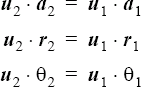For example,
PERIODIC_BOUNDARY_CONDITION( "a pie cut axisymmetric constraint" ) {
variable      = all
type          = axisymmetric
nodal_pairs   = { 1, 1, 2 ; 2, 11, 12 ; 3, 21, 22 ; }
rotation_axis = { 0, 0, 0 ; 0, 0, 1 ; }
}

defines an axis of rotation in the global z-direction. The rotated velocity vectors of nodes 1 and 2 are equal. Similar conditions apply to nodal pairs (11,12) and (21,22). For a scalar variable the axisymmetric periodic boundary condition type is equivalent to the periodic type.

A two_reference_frames periodic boundary condition type is used to interface two regions with different rotating frames of reference that are specified through the ROTATION_FORCE command. This type is obsolete and support for it will be removed eventually. See the REFERENCE_FRAME command for the preferred, and much easier, way of specifying rotating forces and reference frames. At the interface boundaries, there must be two sets of nodes with the same nodal coordinates. Each set belongs to one of the rotating frames of reference. The assignment is implicitly specified by the reference frame of the elements referencing the nodes. For example, consider the case where one element set is rotating in the y-direction, while the other is stationary:
COORDINATE {
coordinates      = { 1, 1.,
0., 0. ;
11, 1., 0., 0. ; # same as node 1
... }
}
ROTATION_FORCE( "rotating frame" ) {
centrifugal       = on
coriolis          = on
center            = { 0, 0, 0 }
angular_velocity  = { 0, 1, 0 }
}
BODY_FORCE( "my body force" ) {
rotation_force    = "rotating frame"
}
ELEMENT_SET( "fluid in rotating frame of reference" ) {
elements          = { 1, 1, 2, 3, 4 ;
... }
body_force        = "my body force"
...
}
ELEMENT_SET( "fluid in stationary frame of reference" ) {
elements          = { 1, 11, 45, 46, 47 ;
... }
body_force        = none
...
}
PERIODIC_BOUNDARY_CONDITION( "interface between two frames of reference" ) {
variable          = all
type              = two_reference_frames
nodal_pairs       = { 1, 1, 11 ;
... }
rotation_force_1  = "rotating frame"
rotation_force_2  = none
}

Nodes one and 11 have the same nodal coordinates. Node one is referenced by the element set "fluid in rotating frame of reference" which is in a rotating frame of reference given by "rotating frame"; and node 11 is referenced by the element set "fluid in stationary frame of reference" which is stationary. The given two_reference_frames periodic boundary condition specifies that the velocities of nodes 1 and 11 transformed to the global stationary frame of reference are equal.

Let u1 and u2 , respectively, be the velocities of the first and second nodes. These node must have common coordinates, denoted here by x. Also, let these nodes be in frames of reference rotating at $\omega$ 1 and $\omega$ 2 radians per unit time and centered at 01 and 02, respectively. Then the velocity periodic boundary condition reduces to:(2)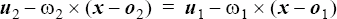For all other variables, the two_reference_frames periodic boundary condition reduces to the simple equality condition. If n > 2 regions with different frames of reference intersect at a point or an edge, one set of nodes for each region is needed and at least n - 1 conditions must be specified. Depending on the particular problem, there are typically several geometrical constraints on the relationship between the parameters of the reference frames and the surface separating the element sets associated with each reference frame. For instance, the velocity at x due to the reference frame motion itself, the second term on each side in the equation above, may need to be parallel to this surface. Since these constraints are not general, AcuSolve cannot check for them and so the responsibility for ensuring geometric compatibility between reference frames falls to you.

A constant_offset periodic boundary condition specifies that the nodal values of the second set are equal to those of the first set plus a constant value. For example,
PERIODIC_BOUNDARY_CONDITION( "inflow/outflow pressure drop" ) {
variable       = pressure
type           = constant_offset
nodal_pairs    = { 1, 101, 201 ; 2, 102, 202 ; 3, 103, 203 ; }
constant_value = 2
}
The pressure of node 201 equals to those of node 101 plus two. That is, let p1 and p2 be the pressure of first and second nodes of a nodal pair, and let c be the constant offset. Then the periodic condition is(3)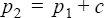For vector variables, constant_values is used to define the offset for each of the three components. For example,
PERIODIC_BOUNDARY_CONDITION( "accelerated velocity" ) {
variable         = velocity
type             = constant_offset
nodal_pairs      = { 1, 15, 16 ; 2, 25, 26 ; }
constant_values  = { 1, 0, 0 }
}
The nodal_pair_offset periodic boundary condition specifies a different offset for each nodal pair. For example,
PERIODIC_BOUNDARY_CONDITION( "interface between two frames of reference" ) {
variable           = velocity
type               = constant_pair_offset
nodal_pairs        = { 1, 1, 11 ; ... }
nodal_pairs_values = { 1, 0, 0, 1 ; ... }
}

This example implements the two_reference_frames periodic boundary condition example given above. The nodal_pair_values parameter is a multi-column array, corresponding to the nodal-pair number and the offset values. For a scalar variable this array has two columns and for a vector variable it has four columns.

The nodal-pair values may be read from a file. For the above example, the values may be placed in a file, such as "two-frame.pbc.val:
1 0 0 1
...
PERIODIC_BOUNDARY_CONDITION( "interface between two frames of reference" ) {
variable           = velocity
type               = constant_pair_offset
nodal_pairs        = { 1, 1, 11 ; ... }
}

A periodic boundary condition of user_function_offset type may be used to model more complex behaviors; see the AcuSolve User-Defined Functions Manual for a detailed description of user-defined functions.

For example, consider the above two reference frame periodic boundary condition. Then the input command may be given by:
PERIODIC_BOUNDARY_CONDITION( "UDF BC for two frames of reference" ) {
variable       = velocity
type           = user_function_offset
nodal_pairs    = { 1, 1, 11 ; ... }
user_function  = "usrPbcOffsetExample"
user_values    = { 0, 0, 0,  # rotating center
1 0, 1, 0,  # angular velocity
1 0, 0, 0,  # rotating center
2 0, 0, 0 } # angular velocity 2
}
where the user-defined function "usrPbcOffsetExample" may be implemented as follows.
#include "acusim.h"
#include "udf.h"
UDF_PROTOTYPE( usrPbcOffsetExample ) ;                 /* function prototype */
Void usrPbcOffsetExample (
UdfHd       udfHd,                                 /* Opaque handle for accessing data   */
Real*       outVec,                                /* Output vector  */
Integer     nItems,                                /* Number of PBC pairs  */
Integer     vecDim                                 /* = 3  */
) {
Integer     pair ;                                 /* a nodal pair counter   */
Real        xOrg1 ;                                /* x-center 1  */
Real        yOrg1 ;                                /* y-center 1  */
Real        zOrg1 ;                                /* z-center 1  */
Real        xOmega1 ;                              /* x-angular velocity 1  */
Real        yOmega1 ;                              /* y-angular velocity 1  */
Real        zOmega1 ;                              /* z-angular velocity 1  */
Real        xOrg2 ;                                /* x-center 2  */
Real        yOrg2 ;                                /* y-center 2   */
Real        zOrg2 ;                                /* z-center 2  */
Real        xOmega2 ;                              /* x-angular velocity 2  */
Real        yOmega2 ;                              /* y-angular velocity 2  */
Real        zOmega2 ;                              /* z-angular velocity 2  */
Real        xOff ;                                 /* reduced x-offset  */
Real        yOff ;                                 /* reduced y-offset  */
Real        zOff ;                                 /* reduced z-offset  */
Real        xOmega ;                               /* reduced x-angular velocity  */
Real        yOmega ;                               /* reduced y-angular velocity  */
Real        zOmega ;                               /* reduced z-angular velocity  */
Real *      crd ;                                  /* coordinates  */
Real *      usrVals ;                              /* user values  */
Real *      xCrd ;                                 /* x-coordinates of node 1 */
Real *      yCrd ;                                 /* y-coordinates of node 1  */
Real *      zCrd ;                                 /* z-coordinates of node 1  */
Real *      xOffset ;                              /* x-component of offset  */
Real *      yOffset ;                              /* y-component of offset  */
Real *      zOffset ;                              /* z-component of offset  */
udfCheckNumUsrVals( udfHd, 12 ) ;                      /* check for error   */
usrVals    = udfGetUsrVals( udfHd ) ;             /* get the user vals  */
xOrg1      = usrVals[ 0] ;                        /* get x-center 1  */
yOrg1      = usrVals[ 1] ;                        /* get y-center 1  */
zOrg1      = usrVals[ 2] ;                        /* get z-center 1  */
xOmega1    = usrVals[ 3] ;                        /* get x-ang vel 1  */
yOmega1    = usrVals[ 4] ;                        /* get y-ang vel 1  */
zOmega1    = usrVals[ 5] ;                        /* get z-ang vel 1  */
xOrg2      = usrVals[ 6] ;                        /* get x-center 2  */
yOrg2      = usrVals[ 7] ;                        /* get y-center 2  */
zOrg2      = usrVals[ 8] ;                        /* get z-center 2  */
xOmega2    = usrVals[ 9] ;                        /* get x-ang vel 2  */
yOmega2    = usrVals[ 10] ;                       /* get y-ang vel 2  */
zOmega2    = usrVals[ 11] ;                       /* get z-ang vel 2  */
xOmega     = xOmega2 - xOmega1 ;                  /* get reduced omega  */
yOmega     = yOmega2 - yOmega1 ;                  /* get reduced omega  */
zOmega     = zOmega2 - zOmega1 ;                  /* get reduced omega  */
xOff       = yOmega2 * zOrg2 - zOmega2 * yOrg2
- yOmega1 * zOrg1 + zOmega1 * yOrg1;   /* get reduced offset  */
yoff       = zOmega2 * xOrg2 - xOmega2 * zOrg2
-  zOmega1 * xOrg1 + xOmega1 * zOrg1;  /* get reduced offset  */
zoff       = xOmega2 * yOrg2 - yOmega2 * xOrg2
- xOmega1 * yOrg1 + yOmega1 * xOrg1    /* get reduced offset  */
crd         = udfGetPbcCrd( udfHd ) ;              /* get the coord.  */
xCrd        = &crd[0*nItems] ;                     /* localize x-coord.  */
yCrd        = &crd[1*nItems] ;                     /* localize y-coord.  */
zCrd        = &crd[2*nItems] ;                     /* localize z-coord.  */
xOffset     = &outVec[0*nItems] ;                  /* localize x-offset  */
yOffset     = &outVec[1*nItems] ;                  /* localize y-offset   */
zOffset     = &outVec[2*nItems] ;                  /* localize z-offset  */
for ( pair = 0 ; pair < nItems ; pair++ ) {
xOffset[pair] = yOmega * zCrd[pair] - zOmega * yCrd[pair] - xOff ;
yOffset[pair] = zOmega * xCrd[pair] - xOmega * zCrd[pair] - yOff ;
zOffset[pair] = xOmega * yCrd[pair] - yOmega * xCrd[pair] - zOff ;
}
} /* end of usrPbcOffsetExample() */

For a vector variable (as above) the dimension of the returned boundary condition vector, outVec, is the number of nodal pairs times three. For a scalar variable, it is the number of nodal pairs.

A constant_coefficients periodic boundary condition specifies a general two-node constraint on a variable. For example,
PERIODIC_BOUNDARY_CONDITION( "inflow/outflow scaled temperature profile" ) {
variable        = temperature
type            = constant_coefficients
nodal_pairs     = { 1, 101, 201 ;
2, 102, 202 ;
3, 103, 203 ; }
constant_values = { 2, -1, 0 }
}
The temperature of node 201 is twice that of node 101. That is, let T1 and T2 be the temperature of first and second nodes of a nodal pair, and let {a1, a2, c} be the coefficients given by constant_values, which is a three-component array in this case. Then the periodic condition is: (4)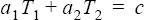For vector variables the constant_values parameter has 21 components, corresponding to three constraints and seven coefficients per constraint. The coefficient are for the x, y, and z components of nodes one and two plus the constraint constant. Let u1, v1, w1, u2, v2, and w2 be the velocity components of the first and second nodes of a pair, and let ${a}_{1}^{1},...,{a}_{6}^{1},{c}^{1},{a}_{1}^{2},...{a}_{6}^{2},{c}^{2},{a}_{1}^{3},...{a}_{6}^{3},{c}^{3}$ be the 21 coefficients given by constant_values. Then the periodic conditions are:(5)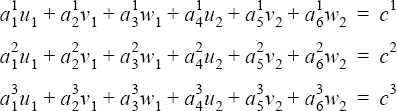The nodal_pair_coefficients periodic boundary condition specifies a different set of coefficients for each pair. For example, let node one and two be at coordinates (1,1,0) and (-1,1,0), then the above axisymmetric periodic boundary condition may be specified by:
PERIODIC_BOUNDARY_CONDITION( "a pie cut axisymmetric constraint" ) {
variable          = velocity
type              = nodal_pair_coefficients
nodal_pairs       = { 1, 1, 2 ;
... }
nodal_pair_values = { 1,  0, 0,  1,  0,  0, -1,   0,  # z-axis
1,  1, 0,  1, -1,  0,  0,       # radial
1, -1, 0, -1, -1,  0,  0;       # tangential... }
}

The nodal_pair_values parameter is a multi-column array, corresponding to the nodal-pair number and the coefficient values. For a scalar variable this array has four columns and for a vector variable it has 22 columns. The nodal-pair values may be read from a file, as given in an example above.

A periodic boundary condition of user_function_coefficients type may be used to model more complex behaviors; see the AcuSolve User-Defined Functions Manual for a detailed description of user-defined functions.

For example, consider a thermal periodic condition where the inflow temperature scaled by the inflow bulk temperature is equal to the outflow temperature scaled by the outflow bulk temperature. Let "inflow surface" and "outflow surface" be the names of SURFACE_OUTPUT sets for inflow and outflow surfaces. Then the input command may be given by:
PERIODIC_BOUNDARY_CONDITION( "UDF BC with fixed wall temperature" ) {
variable       = temperature
type           = user_function_coefficients
user_function  = "usrPbcCoefExample"
user_values    = { 0,    # fixed wall temperature
10,    # initial inflow bulk temperature
9,    # initial outflow bulk temperature
1005 }   # specific heat
user_strings   = { "inflow surface", "outflow surface" }
}
where the user-defined function "usrPbcCoefExample" may be implemented as follows:
#include "acusim.h"
#include "udf.h"
UDF_PROTOTYPE( usrPbcOffsetExample ) ; /* function prototype*/
Void usrPbcOffsetExample (
UdfHd            udfHd,                                /* Opaque handle for accessing data */
Real*            outVec,                               /* Output vector  */
Integer          nItems,                               /* Number of PBC pairs  */
Integer          vecDim                                /* = 3  */
) {
Integer          pair ;                                /* a running index  */
Real             wallTemp ;                            /* wall temperature  */
Real             inBulkTemp ;                          /* inflow bulk temperature  */
Real             outBulkTemp ;                         /* outflow bulk temperature  */
Real             cp ;                                  /* specific heat  */
Real             inCoef ;                              /* inflow temp coefficient  */
Real             outCoef ;                             /* outflow temp coefficient  */
Real             cCoef ;                               /* constant coefficient  */
Real *           convFlux ;                            /* convective temperature flux  */
Real *           massFlux ;                            /* mass flux  */
Real *           usrVals ;                             /* user values  */
Real *           inCoefs ;                             /* return inflow temp coefficient  */
Real *           outCoefs ;                            /* return outflow temp coefficient  */
Real *           cCoefs ;                              /* return constant coefficient  */
String*          usrStrs ;                             /* user strings  */
String           inflowOsi ;                           /* inflow surface output  */
String           outflowOsi ;                          /* outflow surface output */
udfCheckNumUsrVals( udfHd, 4 ) ;                           /* check for error  */
udfCheckNumUsrVals( udfHd, 2 ) ;                           /* check for error  */
usrVals          = udfGetUsrVals( udfHd ) ;            /* get the user vals  */
usrStrs          = udfGetUsrStrs( udfHd ) ;            /* get the user strs  */
wallTemp         = usrVals ;                        /* get wall temp.  */
inBulkTemp       = usrVals ;                        /* get inflow bulk T  */
outBulkTemp      = usrVals ;                        /* get output bulk T */
cp               = usrVals ;                        /* get specific heat  */
inflowOsi        = usrStrs ;                        /* get inflow surf.  */
outflowOsi       = usrStrs ;                        /* get outflow surf.  */
if ( !udfFirstStep(udfHd) ) {                          /* initial step? */
massFlux     = udfGetOsiData( udfHd, inflowOsi,    */
UDF_OSI_MASS_FLUX ) ;               /* inflow mass flux */
convFlux     = udfGetOsiData( udfHd, inflowOsi,
UDF_OSI_CONVECTIVE_TEMPERATURE) ;   /* inflow conv.temp  */
inBulkTemp   = *convFlux / (*massFlux * cp) ;       /* inflow bulk temp  */
massFlux     = udfGetOsiData( udfHd, outflowOsi,
UDF_OSI_MASS_FLUX ) ;               /* outflow mass flux  */
convFlux     = udfGetOsiData( udfHd, outflowOsi,
UDF_OSI_CONVECTIVE_TEMPERATURE) ;   /* outflow conv.temp  */
outBulkTemp  = *convFlux / (*massFlux * cp) ;       /* outflow bulk temp  */
}
if ( inBulkTemp  == wallTemp ) {
udfSetError( udfHd, "inflow bulk temp. equals wall temp." ) ;
}
if ( outBulkTemp == wallTemp ) {
udfSetError( udfHd, "outflow bulk temp. equals wall temp." ) ;
}
inCoef           = 1. / (inBulkTemp - wallTemp) ;
outCoef          = -1. / (outBulkTemp - wallTemp) ;
cCoef            = (inCoef + outCoef) * wallTemp ;
inCoefs          = &outVec[0*nItems] ;                 /* localize outVec  */
outCoefs         = &outVec[1*nItems] ;                 /* localize outVec  */
cCoefs           = &outVec[2*nItems] ;                 /* localize outVec  */
for ( pair = 0 ; pair < nItems ; pair++ ) {
inCoefs [pair]  = inCoef ;
outCoefs[pair]  = outCoef ;
cCoefs [pair]   = cCoef ;
}
} /* end of usrPbcCoefExample() */

The dimension of the returned boundary condition vector, outVec, is the number of nodal pairs times three for this scalar variable. For a vector variable, it is the number of nodal pairs times 21.

The multiplier_function parameter may be used to uniformly scale the offset values for the offset- and coefficient-type periodic boundary conditions. The value of this parameter refers to the user-given name of a MULTIPLIER_FUNCTION command in the input file. For example, to linearly increase the pressure drop between the inflow and outflow nodes, you may specify:
PERIODIC_BOUNDARY_CONDITION( "inflow/outflow pressure drop" ) {
variable             = pressure
type                 = constant_offset
nodal_pairs          = { 1, 101, 201 ;
2, 102, 202 ;
3, 103, 203 ; }
constant_value       = 2
multiplier_function  = "ramped"
}
MULTIPLIER_FUNCTION( "ramped" ) {
type                 = piecewise_linear
curve_fit_values     = { 0, 0 ; 10, 1 }
curve_fit_variable   = time
}
In some circumstances it is necessary to "turn off" or "deactivate" a previously-defined periodic boundary condition. This is accomplished by setting the active flag through active_type. A value of all is the default and means that all of the nodal pairs in the set are active (active flag = 1) and will have the periodic boundary condition imposed on them. A value of none means that no pair is active (active flag = 0). For example, assume that one run was made with the following command to model a periodic channel flow:
PERIODIC_BOUNDARY_CONDITION( "inflow/outflow periodicity" ) {
variable    = all
type        = periodic
}
Then the entire input file to convert this model on restart to a channel with plug flow at the inlet may be given by (assuming the normal to the inlet surface is in the x direction):
RESTART {}
PERIODIC_BOUNDARY_CONDITION( "inflow/outflow periodicity" )
active_type     = none
}
NODAL_BOUNDARY_CONDITION( "inflow x-velocity" ) {
variable        = x_velocity
type            = constant
constant_value  = 1
}
NODAL_BOUNDARY_CONDITION( "inflow y-velocity" ) {
variable        = y_velocity
type            = zero
}
NODAL_BOUNDARY_CONDITION( "inflow z-velocity" ) {
variable        = z_velocity
type            = zero
}
RUN {}

An active flag of user_function type may be used to model more complex behaviors; see the AcuSolve User- Defined Functions Manual for a detailed description of user-defined functions. Values of the active flag between zero and one are allowed; such values are used to linearly interpolate between the previous value of the periodic condition and the given condition, with the result imposed as the actual periodic condition.

For example, consider a model of a window suddenly opening. Two surfaces with coincident nodes define the window. When it is closed, all the nodes have no-slip velocity boundary conditions and no condition on the pressure, which allows the window to support a pressure difference. When the window opens, the no-slip conditions are removed by turning the active flag off in the NODAL_BOUNDARY_CONDITION commands and the pairs of coincident nodes are made equivalent by applying a periodic boundary condition on all variables, turning on the active flag. The input commands for implementing this model may be given by:
NODAL_BOUNDARY_CONDITION( "UDF opening window for x-velocity" ) {
variable             = x_velocity
type                 = zero
active_type          = user_defined
active_user_function = "usrActiveFlagNBC"
active_user_values   = { 100,    # deactivation time
0.1 } # mushy interval for time
}
PERIODIC_BOUNDARY_CONDITION( "UDF opening window" ) {
variable             = all
type                 = periodic
active_type          = user_defined
active_user_function = "usrActiveFlagNBC"
active_user_values   = { 100,    # deactivation time
0.1 } # mushy interval for time
}
with similar commands for the y- and z-velocities. The user-defined function "usrActiveFlagPBC" may be implemented as follows.
#include "acusim.h"
#include "udf.h"
UDF_PROTOTYPE( usrActiveFlagPBC ) ;          /* function prototype */
Void usrActiveFlagPBC (
UdfHd        udfHd,                      /* Opaque handle for accessing data  */
Real*        outVec,                     /* Output vector  */
Integer      nItems,                     /* Number of PBC pairs  */
Integer      vecDim                      /* = 1  */
) {
Integer      pair ;                      /* nodal pair counter  */
Real         t0 ;                        /* activation time   */
Real         mushy ;                     /* mushy interval in time  */
Real         delta_t ;                   /* time relative to activation time  */
Real         time ;                      /* time  */
Real*        usrVals ;                   /* user values  */
udfCheckNumUsrVals( udfHd, 2 ) ;             /* check for error       */
time         = udfGetTime( udfHd ) ;     /* get the time  */
usrVals      = udfGetUsrVals( udfHd ) ;  /* get the user vals  */
t0           = usrVals ;              /* get activation time  */
mushy        = usrVals ;              /* get mushy interval  */
for ( pair = 0 ; pair < nItems ; pair++ ) {
delta_t = time - t0 ;
if ( delta_t >= mushy ) {
outVec[pair] = 1 ;
} else if ( delta_t <= 0 ) {
outVec[pair] = 0 ;
} else {
outVec[pair] = delta_t / mushy ;
}
}
} /* end of usrActiveFlagPBC() */

The dimension of the returned active flag vector, outVec, is the number of nodal pairs. The implementation for the user-defined function "usrActiveFlagNBC" is similar except the activation flag changes from one to zero at the activation time. See NODAL_BOUNDARY_CONDITION for an example.